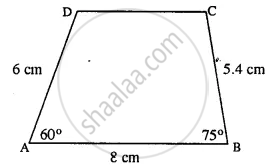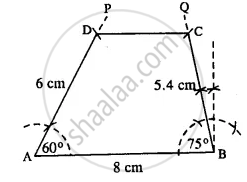# Construct a Quadrilateral Abcd; If: Ab = 8 Cm, Bc = 5.4 Cm, Ad = 6 Cm, ∠A = 60° and ∠B = 75°. - Mathematics

Sum

AB = 8 cm, BC = 5.4 cm, AD = 6 cm, ∠A = 60° and ∠B = 75°.

#### Solution

The rough figure is as follow :The actual quadrilateral is constructed with the help of the above rough figure.Steps :

1. Draw AB = 8 cm.
2. At A, draw ∠PAB = 60° and cut DA = 6 cm.
3. At B, draw ∠QBA = 75° and cut BC = 5.4 cm.
4. Join DC.
Thus ABCD is the required quadrilateral.
Is there an error in this question or solution?

#### APPEARS IN

Selina Concise Mathematics Class 8 ICSE
Chapter 18 Constructions
Exercise 18 (D) | Q 1.3 | Page 211